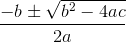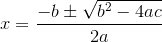# How to understand math formulasBy Murray Bourne, 19 Feb 2009

In a recent IntMath Poll, many readers reported that they find math difficult because they have trouble learning math formulas and an almost equal number have trouble understanding math formulas.

I wrote some tips on learning math formulas here: How to learn math formulas.

Now for some suggestions on how to understand math formulas. These should be read together with the “learning” tips because they are closely related.

a. Understanding math is like understanding a foreign language: Say you are a native English speaker and you come across a Japanese newspaper for the first time. All the squiggles look very strange and you find you don’t understand anything.

If you want to learn to read Japanese, you need to learn new symbols, new words and new grammar. You will only start to understand Japanese newspapers (or manga comics ^_^) once you have committed to memory a few hundred symbols & several hundred words, and you have a reasonable understanding of Japanese grammar.

When it comes to math, you also need to learn new symbols (like π, θ, Σ), new words (math formulas & math terms like “function” and “derivative”) and new grammar (writing equations in a logical and consistent manner).

So before you can understand math formulas you need to learn what each of the symbols are and what they mean (including the letters). You also need to concentrate on the new vocabulary (look it up in a math dictionary for a second opinion). Also take note of the “math grammar” — the way that it is written and how one step follows another.

A little bit of effort on learning the basics will produce huge benefits.

b. Learn the formulas you already understand: All math requires earlier math. That is, all the new things you are learning now depend on what you learned last week, last semester, last year and all the way back to the numbers you learned as a little kid.

If you learn formulas as you go, it will help you to understand what’s going on in the new stuff you are studying. You will better recognize formulas, especially when the letters or the notation are changed in small ways.

Don’t always rely on formula sheets. Commit as many formulas as you can to memory — you’ll be amazed how much more confident you become and how much better you’ll understand each new concept.

c. Always learn what the formula will give you and the conditions: I notice that a lot of students write the quadratic formula asBut this is NOT the quadratic formula! Well, it’s not the whole story. A lot of important stuff is missing — the bits which help you to understand it and apply it. We need to have all of the following when writing the quadratic formula:

The solution for the quadratic equation

ax2 + bx + c = 0

is given byA lot of students miss out the “x =” and have no idea what the formula is doing for them. Also, if you miss out the following bit, you won’t know how and when to apply the formula:

ax2 + bx + c = 0

Learning the full situation (the complete formula and its conditions) is vital for understanding.

d. Keep a chart of the formulas you need to know: Repetition is key to learning. If the only time you see your math formulas is when you open your textbook, there is a good chance they will be unfamiliar and you will need to start from scratch each time.

Write the formulas down and write them often. Use Post-It notes or a big piece of paper and put the formulas around your bedroom, the kitchen and the bathroom. Include the conditions for each formula and a description (in words, or a graph, or a picture).

The more familiar they are, the more chance you will recognize them and the better you will understand them as you are using them.

e. Math is often written in different ways — but with the same meaning: A lot of confusion occurs in math because of the way it is written. It often happens that you think you know and understand a formula and then you’ll see it written in another way — and panic.

A simple example is the fraction “half”. It can be written as 1/2, and also diagonally, as ½ and in a vertical arrangement like a normal fraction. We can even have it as a ratio, where the ratio of the 2 (equal) parts would be written 1:1.

Another example where the same concept can be written in different ways is angles, which can be written as capital letters (A), or maybe in the form ∠BAC, as Greek letters (like θ) or as lower case letters (x). When you are familiar with all the different ways of writing formulas and concepts, you will be able to understand them better.

Every time your teacher starts a new topic, take particular note of the way the formula is presented and the alternatives that are possible.

Do you have any tips to add? How do you figure out your math formulas? Which formulas are hardest to understand?

Good luck with understanding math formulas!

How to learn math formulas

Ten Ways to Survive the Math Blues

How to get better at math,
How to get good at math,
why is math so hard,8th grade math tutoring,kids math tutor,math tutor for kids,7th grade math tutoring,math tutoring for kids,
math apps for kids,
free math websites, how to get better at math fast,how to become good at math overnight,
math made easy,how to understand maths easily,
how to get better at math for adults,
how to become good at math overnight,
how to be good at math,
how to get good at math again,
how to get good at math reddit,
how to understand maths easily,
math games,